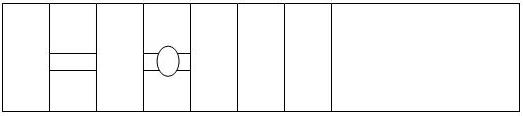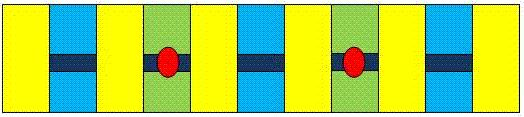Courses

# CBSE Class 3 Maths Worksheet- Shapes And Designs Notes | EduRev

## Class 3 : CBSE Class 3 Maths Worksheet- Shapes And Designs Notes | EduRev

The document CBSE Class 3 Maths Worksheet- Shapes And Designs Notes | EduRev is a part of the Class 3 Course Mathematics for Class 3: NCERT.
All you need of Class 3 at this link: Class 3

Q.1. What types of surfaces do the following objects have?
(i) Brinjal (long)
(ii) Elephant
(iii) Table
Ans.
(i) Cylindrical
(ii) Curved
(iii) Plane

Q.2. Name the types of surfaces that you know.
Ans.
Plane surface, curved surface, cylindrical.

Q.3. Name five solid shapes.
Ans.
Cube, Cuboid, Cylinder, Cone and Sphere.

Q.4. Write down the number of faces, edges and vertices of the following:
(i) Cuboid
(ii) Cylinder
(iii) Sphere
Ans.
(i) Cuboid has 6 faces, 12 edges and 8 vertices.
(ii) Cylinder has 3 faces, 2 edges and no vertices.
(iii) Sphere has 1 face (curved), no edges and no vertex.

Q.5. Write the number of sides and corners of the following:
(i) Square
(ii) Triangle
(iii) Circle
Ans.
(i) A square has four sides and four corners
(ii) A triangle has three sides and three corners
(iii) A circle has one side (circumference) and no corners

Q.6. Follow the pattern and form a design then color it on your own choice to look the design colorful.Ans.Q.7. Write ‘true’ or ‘false’ for each statement:
(i) A square has 4 sides and 4 vertices.
(ii) All the edges of a cuboid are equal.
(iii) All the edges of a cube are not equal.
(iv) A circle has a centre and a circumference.
(v) Only one line can pass through a point.
(vi) The diameter of a circle is double the length of its radius.
Ans.
(i) True
(ii) False
(iii) False
(iv) True
(v) False
(vi) True

Offer running on EduRev: Apply code STAYHOME200 to get INR 200 off on our premium plan EduRev Infinity!

## Mathematics for Class 3: NCERT

56 videos|42 docs|44 tests

,

,

,

,

,

,

,

,

,

,

,

,

,

,

,

,

,

,

,

,

,

;